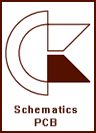# Amiga Models

A500 Rev. 6 (R1.37)A600 Rev. 1.5 (R1.37)A600 Rev. 2 (R1.37)A1200 Rev. 1.0 (R1.38)A1200 Rev. 2.0 (R1.38)A2000 Rev. 6 (R1.38)A3000 Rev. 9 (R1.38)A3000T Rev. 6.1 (R1.38)A4000 Rev. B (R1.37)A4000 Rev. D (R1.38)A4000T Rev. 4.1 (R1.37)A4000T Module (R1.37)CD32 Rev. 3.0 (R1.43)CD32 Rev. 4.0 (R1.43)# Expansions

A2660 (R1.38)A2065 Rev.2 (R1.38)A2320 Rev.2 (R1.38)A2410 Rev.6 (1.38)A2620 (R1.38)A2630 Rev.9 (R1.38)A3630 (R1.38)A3640 Rev.3.1 (R1.38)